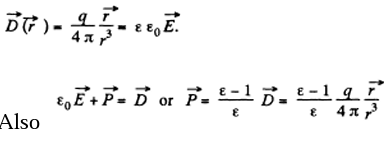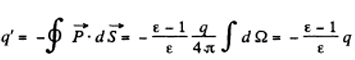# I.E. Irodov Solutions on Conductors and Dielectrics in an Electric Field

Conductors and Dielectrics in an Electric Field is a significant chapter in Physics that mainly deals with the electric field and properties with various materials like conductors and dielectrics. The chapter comprises various numerical based on the syllabus and also the practical applications. I.E. Irodov Physics provides the solutions to different varieties of numerical. Conductors and Dielectrics in an Electric Field explains the importance of its materials in the electrical circuit. is a vital resource element that is needed by all students to score good marks in Class 12 Physics examinations and entrance examinations. It is one of the best ways to study and practice for physics examinations to score high. The problems asked here are mainly based on electric field, current, speed, voltage and so on.

The behaviour of conductors and dielectrics is explained in depth. The problems connect the theoretical concepts with practical and also enhance their applications in daily life and activities. Students striving for a high score in competitive examination must solve all the given problems for easy understanding and to have a grip over the syllabus and concept.

One can get access to all sorts of problems based on each and every formula, equations and method. The solutions to every problem is explained stepwise with related data, formula, graphs and equations. Students can easily get access to all varieties of questions based on conductors and dielectrics. This helps students to make the concept clear and get a thorough understanding.

### I.E. Irodov Solutions on Conductors and Dielectrics in an Electric Field

1. A small ball is suspended over an infinite horizontal conducting plane by means of an insulating elastic thread of stiffness k. As soon as the ball was charged, it descended by x cm and its separation Horn the plane became equal to l. Find the charge of the ball.

Solution:

1. When the ball is charged, for the equilibrium of ball, electric force on it must counterbalance the excess spring force, exerted, on the ball due to the extension in the spring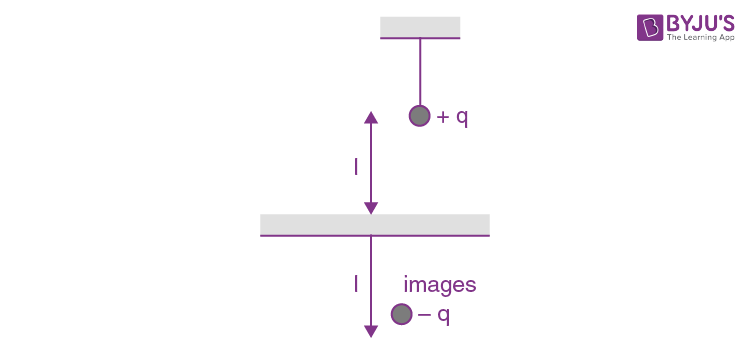Thus, Fd=Fspr

Or

$\frac{q^{2}}{4\pi \varepsilon _{0}(2l)^{2}}=kx$

(The force on the charge q might be considered as arisen from attraction by electrical image)

Or $q= 4l\sqrt{\pi \varepsilon _{o}kx}$

Sought charge on sphere.

2. A point charge q is located at a distance l from the infinite conducting plane. What amount of work has to be performed in order to slowly remove this charge very far from the plane.

Solution:

1. By definition, the work of this force done upon a displacement dx (Fig.) is given by elementary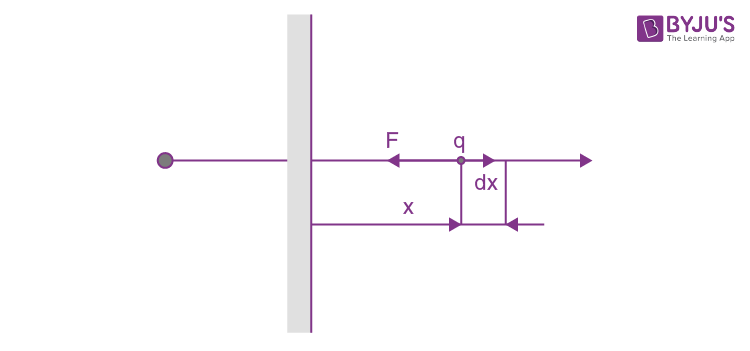$dA =F_{x}dx=-\frac{q^{2}}{4\pi \varepsilon _{o}(2x)^{2}}dx$

Where the expression for the force is obtained with the help of the image method. Integrating this equation over x between l and ∞, we find

$A =-\frac{q^{2}}{16\pi \varepsilon _{o}}\int_{1}^{\infty }\frac{dx}{x^{2}}= \frac{q^{2}}{16\pi \varepsilon _{o}1}$

3. Two-point charges, q and — q, are separated by a distance of 1, both being located at a distance l/2 from the infinite conducting plane. Find:

1. (a) the modulus of the vector of the electric force acting on each charge;

2. (b) the magnitude of the electric field strength vector at the midpoint between these charges.

Solution:

1. Using the concept of electrical image, it is clear that the magnitude of force acting on each charge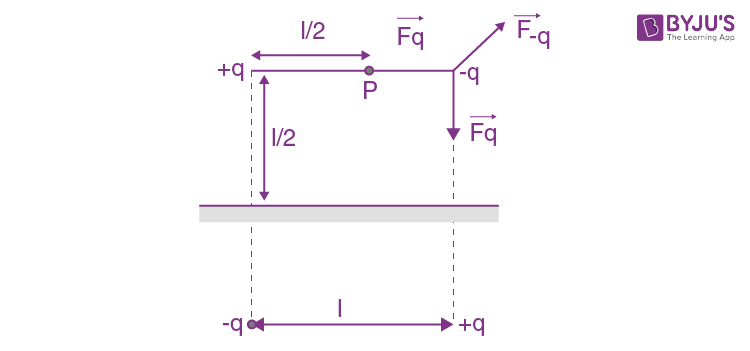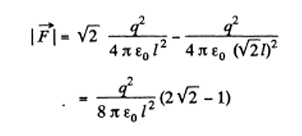(b) Also, from the figure, the magnitude of electric field strength at P

$E= 2\left ( 1- \frac{1}{5\sqrt{5}} \right )\frac{q}{\pi \varepsilon _{o}l^{2}}$

4. A point charge q is located between two mutually perpendicular conducting half-planes. Its distance from each half-plane is equal to l. Find the modulus of the vector of the force acting on the charge.

Solution:

1. Using the concept of electrical image, it is easily seen that the force on the charge q is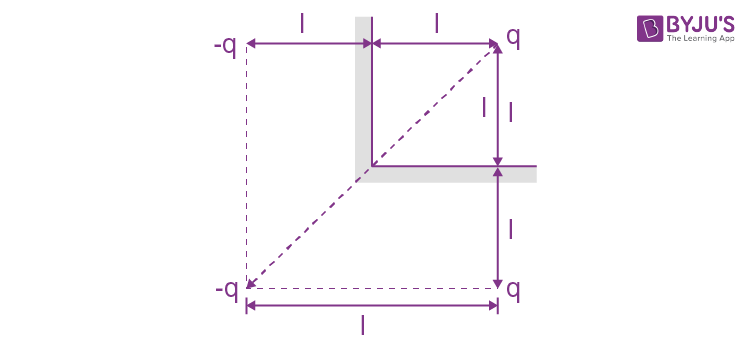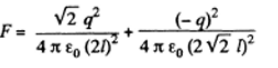$\frac{(2\sqrt{2}-1)q^{2}}{32\pi \varepsilon _{o}l^{2}}$

5. A point dipole with an electric moment p is located at a distance l from an infinite conducting plane. Find the modulus of the vector of the force acting on the dipole if the vector p is perpendicular to the plane.

Solution:

1. Using the concept of electrical image, force on the dipole $\vec{p}$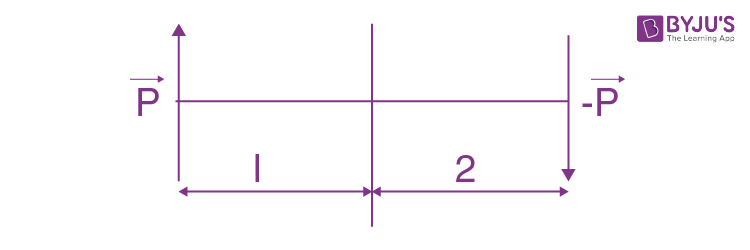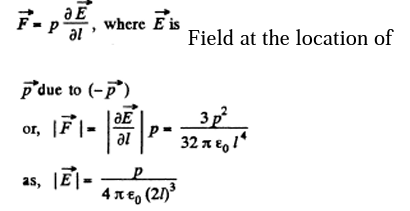6. A point charge q is located at a distance l from an infinite conducting plane. Determine the surface density of charges induced on the plane as a function of separation r from the base of the perpendicular drawn to the plane from the charge.

Solution:

1. To find the surface charge density, we must know the electric field at the point P (Fig.) which is at a distance r from the point O.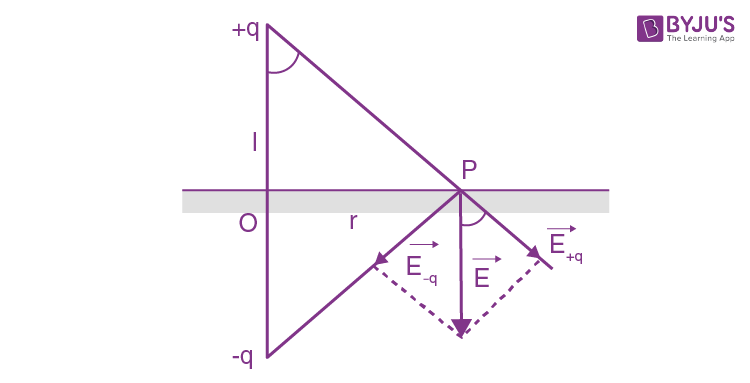Using the image mirror method, the field at P,

$E=2E\cos \alpha =2\frac{q}{4\pi \varepsilon _{o}x^{2}}\frac{l}{x}=\frac{ql}{2\pi \varepsilon _{o}(l^{2}+r^{2})^{\frac{3}{2}}}$

Now from Gauss’ theorem, the surface charge density on the conductor is connected with the electric field near its surface (in vacuum) through the relation

$\sigma =\varepsilon _{o}E_{n}$

Where En is the projection of $\vec{E}$ onto the outward normal $\vec{n}$ with respect to the conductor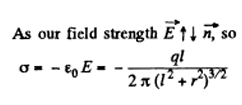7. A thin infinitely long thread carrying a charge λ per unit length is oriented parallel to the infinite conducting plane. The distance between the thread and the plane is equal to l. Find:

1. (a) the modulus of the vector of the force acting on a unit length of the thread;

2. (b) the distribution of surface charge density σ (x) over the plane, where x is the distance from the plane perpendicular to the conducting surface and passing through the thread.

Solution:

1. (a)The force F1

on the unit length of the thread is given by

$F_{1}=\lambda E_{1}$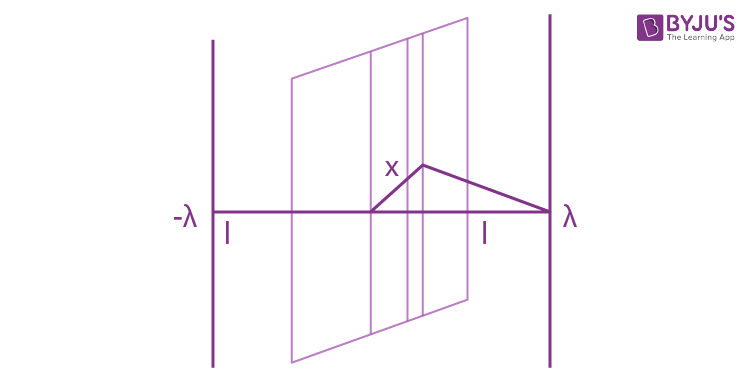Where E1 is the field at the thread due to image charge

$E_{1}=-\frac{\lambda }{2\pi \varepsilon _{o}2l}$

Thus,

$F_{1}=-\frac{\lambda^{2} }{4\pi \varepsilon _{o}l}$

(b)There is an image thread with charge density λ behind the conducting plane. We calculate the electric field on the conductor. It is

$E(x)= E_{n}x=\frac{\lambda l}{\pi \varepsilon _{0}(x^{2}+l^{2})}$

On considering the thread and its image

$\sigma(x)=\varepsilon _{n}E_{n}=\frac{\lambda l}{\pi (x^{2}+ l^{2})}$

8. A very long straight thread is oriented at right angles to an infinite conducting plane; its end is separated from the plane by a distance l. The thread carries a uniform charge of linear density λ. Suppose point 0 is the trace of the thread on the plane. Find the surface density of the induced charge on the plane

1. (a) at the point 0;

2. (b) as a function of a distance r from the point 0.

Solution:

1. (a) At o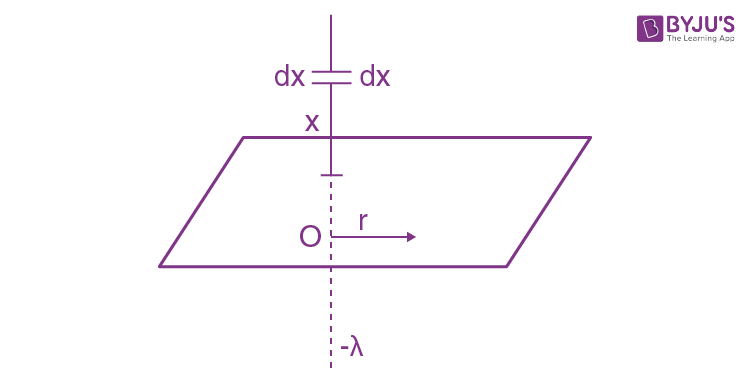(b)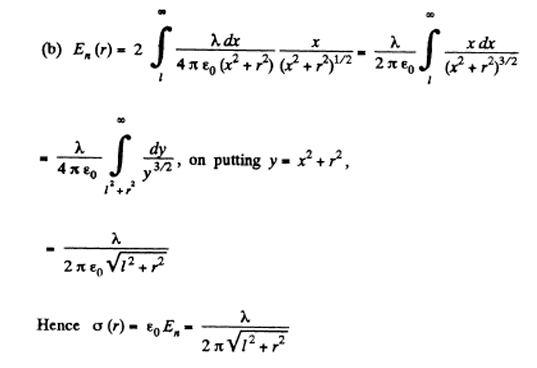9. A thin wire ring of radius R carries a charge q. The ring is oriented parallel to an infinite conducting plane and is separated by a distance l from it. Find:

1. (a) the surface charge density at the point of the plane symmetrical with respect to the ring;

2. (b) the strength and the potential of the electric field at the centre of the ring

Solution:

1. It can be easily seen that in accordance with the image method, a charge q must be located on a similar ring but on the other side of the conducting plane at the same perpendicular distance.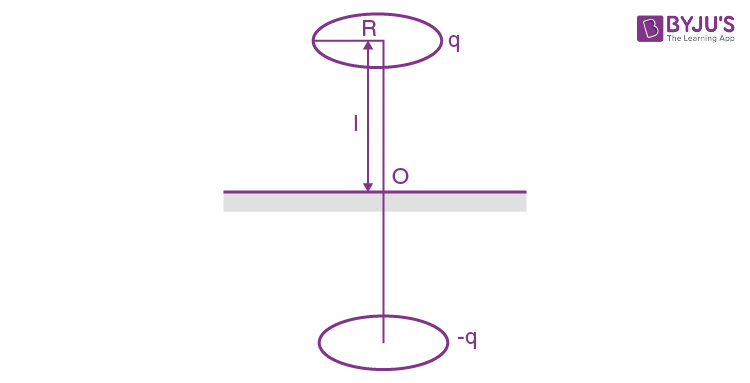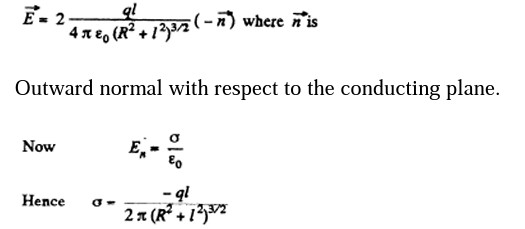Where minus sign indicates that the induced charge is opposite in sign to that of charge q>0.

10. Find the potential ϕ of an uncharged conducting sphere outside of which a point charge q is located at a distance of 1 from the sphere’s centre.

Solution:

1. Potential ϕ the same for all points of the sphere. Thus we calculate its value at the centre O of the sphere. Thus we can calculate its value at the centre O of the sphere because only for this point, it can be calculated in the most simple way

$\phi=\frac{1}{4\pi\varepsilon _{o}}\frac{q}{l} + \phi ^{'}$-------(1)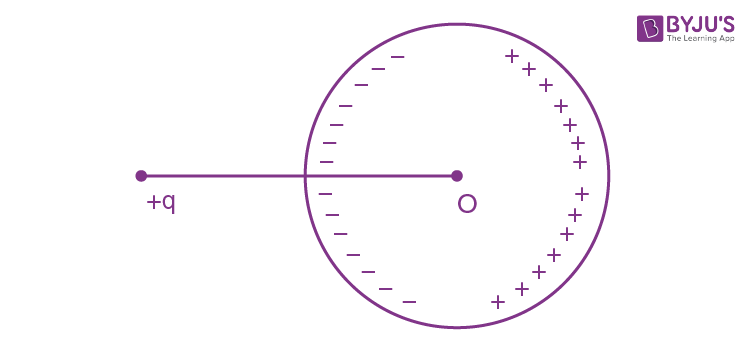Where the first term is the potential of charge q, while the second is the potential due to the charges induced on the surface of the sphere. But since all induced charges are at the same distance equal to the radius of the circle from the point C and the total induced charge is equal to zero, φ' = 0, as well. Thus equation (1) is reduced to the form

$\phi=\frac{1}{4\pi\varepsilon _{o}}\frac{q}{l}$

11. A point charge q is located at a distance r from the centre O of an uncharged conducting spherical layer whose inside and outside radii are equal to

R1 and R2 respectively. Find the potential at the point O if r <R1.

Solution:

1. As the sphere has conducting layers, charge q is induced on the inner surface of the sphere q and consequently, charge +q is induced on the outer layer as the sphere as a whole is uncharged.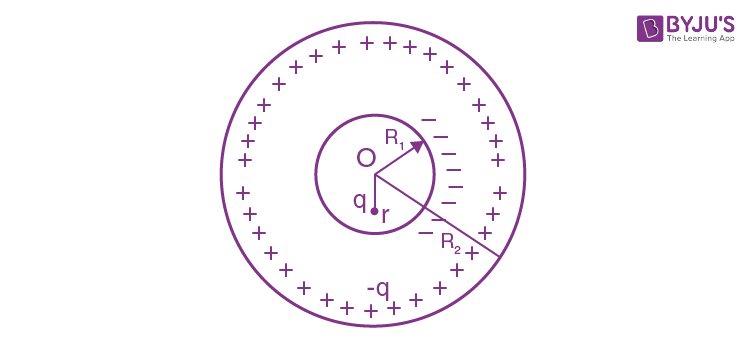Hence, the potential at O is given by,

$\phi_{o}=\frac{q}{4\pi\varepsilon _{o}r}+\frac{-q}{4\pi\varepsilon _{o}R_{1}}+\frac{q}{4\pi\varepsilon _{o}R_{2}}$

It should be noticed that the potential can be found in such a simple way only at O, since all the induced charges are at the same distance from this point, and their distribution, (which is unknown to us), does not play any role.

12. A system consists of two concentric conducting spheres, with the inside sphere of radius a carrying a positive charge q1. What charge q2 has to be deposited on the outside sphere of radius b to reduce the potential of the inside sphere to zero? How does the potential φ depend in this case on a distance r from the centre of the system? Draw the approximate plot of this dependence.

Solution:

1. Potential at the inside sphere

$\\phi_{a}=\frac{q}{4\pi\varepsilon _{o}a}+\frac{q_{2}}{4\pi\varepsilon _{o}b}$

Obviously

$\\phi_{a}=0$ and $q_{2}= -\frac{b}{a}q_{1}$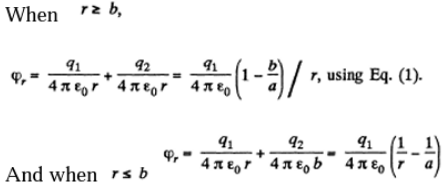13. Four large metal plates are located at a small distance d from one another as shown in Figure. The extreme plates are interconnected by means of a conductor while a potential difference Δφ is applied to internal plates. Find:

1. (a) the values of the electric field strength between neighbouring plates;

2. (b) the total charge per unit area of each plate.

Solution:

1. As the metallic plates 1 and 4 are isolated and connected by means of a conductor, φ1 = φ4. Plates 2 and 3 have the same amount of positive and negative charges and due to induction, plates 1 and 4 are respectively negatively and positively charged and in addition to it all the four plates are located a small but at equal distance d relative to each other, the magnitude of electric field strength between 1 - 2 and 3 - 4 are both equal in magnitude and direction say $\vec{E}$. Let $\vec{E'}$ be the field strength between the plates 2 and 3 which is directed form 2 to 3.

Hence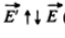According to the question

$E'd=\Delta \phi =\phi _{2}-\phi _{3}$----(i)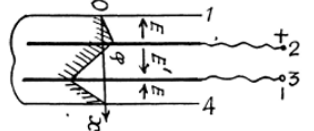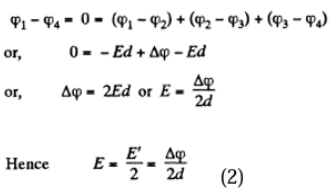(b) Since E α σ, we can state that according to equation (2) for part (a) the charge on the p4th 2 is divided into two parts; such that 1/3rd of it lies on the upper side and 2/3rd

Thus charge density of upper face of plate 2 or of plate 1 or plate 4 and lower face of

$3\sigma =\varepsilon _{0}E=\frac{\varepsilon _{o}\Delta \phi }{2d}$

and charge density of lower face of 2 or upper face of 3

$\sigma' =\varepsilon _{0}E'=\frac{\varepsilon _{o}\Delta \phi }{d}$

Hence the net charge density of plate 2 or 3 becomes

$\sigma +\sigma' =\frac{3\varepsilon _{0}\Delta \phi}{2d}$

14. Two infinite conducting plates 1 and 2 are separated by a distance l. A point charge q is located between the plates at a distance x from plate 1. Find the charges induced on each plate.

Solution:

1. The problem of point charge between two conducting planes is more easily tackled (if we want only the total charge induced on the planes) if we replace the point charge with a uniformly charged plane sheet. Let σ be the charge density on this sheet and E1 and E2 outward electric field on the two sides of the sheet.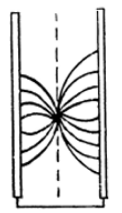Then $E_{1}+ E_{2}= \frac{\sigma }{\varepsilon _{o}}$

The conducting planes will be assumed to be grounded.

$E_{1} x = E_{2}(l-x)$

Hence

$E_{1}=\frac{\sigma}{l\varepsilon _{o}}(l-x)$

And

$E_{2}=\frac{\sigma}{l\varepsilon _{o}}(x)$

This means that the induced charge density on the plane conductors are

$\sigma _{1}=-\frac{\sigma}{l}(l-x)$

$\sigma _{2}=-\frac{\sigma}{l}(x)$

Hence

$q_{1}=-\frac{q}{l}(l-x)$

$q_{2}=-\frac{q}{l}(x)$

15. Find the electric force experienced by a charge reduced to a unit area of an arbitrary conductor if the surface density of the charge equals σ.

Solution:

1. Near the conductor $E=E_{n}=\frac{\sigma }{\varepsilon _{0}}$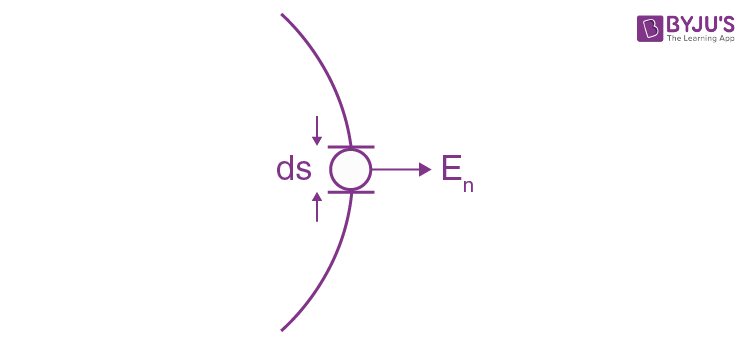This field can be written as the sum of two parts E. The remaining part contributes E1 and E2. E1 is the electric field due to infinitesimal area dS.

$E_{1}=\pm \frac{\sigma }{2\pi \varepsilon _{o}}$

The remaining part contributes $E_{2}=\frac{\sigma }{2\pi \varepsilon _{o}}$ on both sides. In calculating the force on the element dS we drop E 1 (because it is a self force.) Thus

$\frac{dF}{ds}= \sigma .\frac{\sigma }{2\varepsilon_{o}}= \frac{\sigma ^{2}}{2\varepsilon_{o}}$

16. A metal ball of radius R = 1.5 cm has a charge q = 10 μC. Find the modulus of the vector of the resultant force acting on a charge located on one half of the ball.

Solution:

1. The total force on the hemisphere is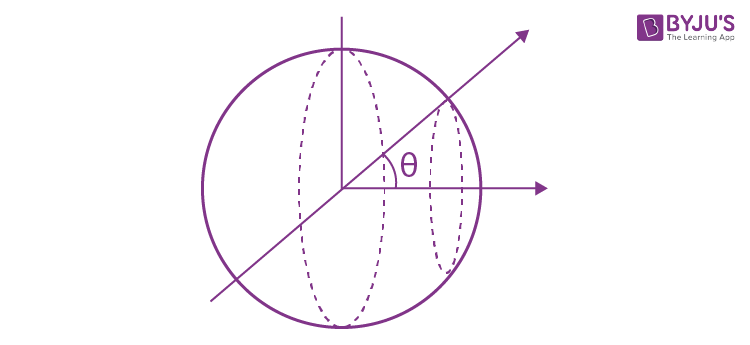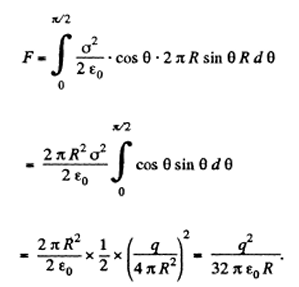17. When an uncharged conducting ball of radius R is placed in an external uniform electric field, a surface charge density σ = σ0cos θ is induced on the ball's surface (here ao is a constant, is a polar angle). Find the magnitude of the resultant electric force acting on an induced charge of the same sign.

Solution:

1. We know that the force acting on the area element dS of a conductor is,

$d\vec{F}=\frac{1}{2}\sigma \vec{E}ds$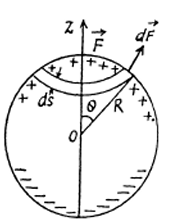It follows from symmetry considerations that $\vec{F}$ is directed along z -axis and hence it can be represented as the sum of the projection of elementary forces (1) onto the z-axis.

dFz=dF cosθ----(2)

Let us consider an elemental area

$ds=2\pi \sin \theta R d\theta$

Considering that

$E=\frac{\sigma }{\varepsilon _{o}}$ equation 2 becomes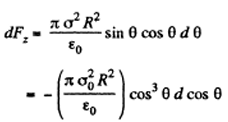Integrating this expression over the half-sphere (i.e.with respect to cos between 0 and 1 )we get

$F=F_{z}=\frac{\pi\sigma _{0}^{2}R^{2} }{4\pi \varepsilon _{0}}$

18. An electric field of strength E = 1.0 kV/cm produces polarization in water equivalent to the correct orientation of only one out of N molecules. Find N. The electric moment of a water molecule equals p = 0.62-10-29 C•m

Solution:

1. The total polarization is $P=(\varepsilon-1)\varepsilon _{0}E$

This must equals $\frac{n_{o}P }{N}$

Where no is the concentration of water molecules

Thus on substituting values we get,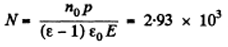19. A nonpolar molecule with polarizability β is located at a great distance l from a polar molecule with electric moment p. Find the magnitude of the interaction force between the molecules if the vector p is oriented along a straight line passing through both molecules.

Solution:

1. From general we know that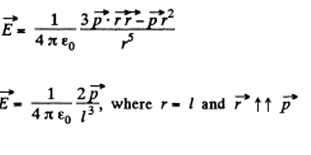This will cause the induction of a dipole moment.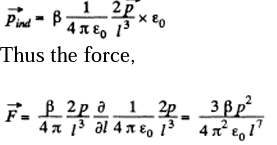20. A nonpolar molecule is located at the axis of a thin uniformly charged ring of radius R. At what distance x from the ring's centre is the magnitude of the force F acting on the given molecule

1. (a) equal to zero;

2. (b) maximum?

Solution:

1. The electric field E at distance x from the centre of the ring is,

$E(x)=\frac{qx}{4\pi \varepsilon _{0}(R^{2}+x^{2})\frac{3}{2}}$

The induced dipole moment is

$p=\beta \varepsilon _{0}E=\frac{q\beta x}{4\pi(R^{2}+x^{2})^{\frac{3}{2}} }$

The force on this molecule is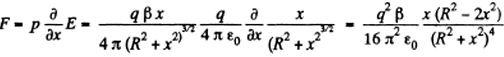This vanishes for

$x=\frac{\pm R}{\sqrt{2}}$

It is maximum when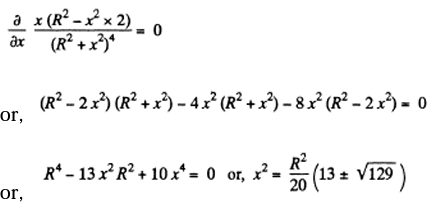$x=\frac{R}{\sqrt{20}}\sqrt{13\pm \sqrt{129}}$

Question 21. A point charge q is located at the centre of a ball made of uniform isotropic dielectric with permittivity ε. Find the polarization P as a function of the radius vector r relative to the centre of the system, as well as the charge q' inside a sphere whose radius is less than the radius of the ball.

Solution

Inside the ball